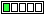All about flooble | fun stuff | Get a free chatterbox | Free JavaScript | Avatarsperplexus dot infoGetting 189 (Posted on 2016-09-05)Evaluate cubes of three consecutive positive integers.
Add up the digits in each of the three results, and add again until you’ve reached a single digit for each of the three numbers.
For example:
46^3 = 97336; 9 + 7 + 3 + 3 + 6 = 28; 2 + 8 = 10; 1 + 0 = 1
47^3 = 103823; 1 + 0 + 3 + 8 + 2 + 3 = 17; 1 + 7 = 8
48^3 = 110592; 1 + 1 + 0 + 5 + 9 + 2 = 18; 1 + 8 = 9

Putting the three digits in ascending order will always give the result 189.

Source:Kendall and Thomas "Mathematical Puzzles for the Connoisseur (1971)"

 No Solution Yet Submitted by Ady TZIDON No RatingComments: ( Back to comment list | You must be logged in to post comments.)solution Comment 1 of 1
The mod-9 values of the cubes of the integers mod 3 have a cycle of 3, so the modular (base 9) values of the cubes depend on only the modular value of the number (mod 3).

1      1
2      8
3      0
4      1
5      8
6      0
7      1
8      8
9      0

This being modular arithmetic, this continues forever.

The three consecutive positive integers will of course have the three different mod-3 values and the cubes will have the same set of three values mod 9: 1, 8 and 0.

The digital root of a number that's 1 mod 9 will be 1; that of a number that's 8 mod 9 will be 8; that of a number that's 0 mod 9 will be 9 (other than zero itself of course). So 1, 8 and 9 will be the digits, and the instructions are to put them in ascending order, so we get 189.

 Posted by Charlie on 2016-09-05 10:05:38Please log in:

 Search: Search body:
Forums (0)